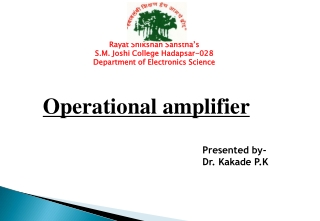DownloadDownload PresentationOperational amplifier

# Operational amplifier

Télécharger la présentation## Operational amplifier

- - - - - - - - - - - - - - - - - - - - - - - - - - - E N D - - - - - - - - - - - - - - - - - - - - - - - - - - -
##### Presentation Transcript

1. RayatShikshanSanstha’s S.M. Joshi College Hadapsar-028 Department of Electronics Science Operational amplifier Presented by- Dr. Kakade P.K

2. The operational amplifier or op-amp is a circuit of components integrated into one chip. A typical op-amp is powered by two dc voltages and has an inverting(-) and a non-inverting input (+) and an output.An op amp is an electronic device which provides a voltage output based on the voltage input introduction

3. Basic op-amp introduction

4. Operational Amplifiers Five important pins • 2 – The inverting input • 3 – The non-inverting input • 6 – The output • 4 – The negative power supply V- (-Vcc) • 7 – The positive power supply V+ (+Vcc) introduction

5. Operational Amplifiers • The output of the op amp is given by the following equation: Vd = E1 – E2 and Vo = AVOL(Vd) • AVOL is called the open-loop voltage gain because it is the gain of the op amp without any external feedback from output to input introduction

6. Operational Amplifiers • Positive Saturation – where the output voltage exceeds the positive power input introduction

7. Operational Amplifiers • Linear Region – where the output voltage is linear based on A (gain) introduction

8. Operational Amplifiers • Negative Saturation – where the output voltage would be less than the negative power input introduction

9. Operational Amplifiers introduction

10. What do they really look like? introduction

11. IC Circuit introduction

12. Operational Amplifiers introduction

13. Operational Amplifiers • An ideal op-amp has infinite gain and bandwidth, we know this is impossible. • However, op-amps do have: • very high gain • very high input impedance(Zin = ∞) • very low output impedance (Zout = 0) • wide bandwidth. introduction

14. Application in op-amp • There are 2 types of application in op-amp • Linear application • Non-linear application • Linear application is where the op-amp operate in linear region: • Assumptions in linear application: • Input current, Ii = 0 • Input voltage: V+=V- • Feedback at the inverting input application

15. Application in op-amp • Non-linear application is where the op-amp operate in non-linear region • By comparing these two input voltages: positive input voltages, V+ and negative input voltage, V- where: VO = VCC if V+ > V- VO = -VEE if V+ < V- • Input current, Ii = 0 application

16. Applications of op-amp • Comparator • Inverter • Audio amplifier • Difference Amplifier • Filter • Summing Amplifier application

17. Op-amp Circuit Application • Inverting Amplifier • Non-Inverting Amplifier • Summing Amplifier • Unity Follower • Difference Amplifier • Integrators • Differentiators application

18. Application: Inverting amplifier • Provide a constant gain multiplier • Input signal is connected to the inverting input of the op-amp. Therefore, the output signal is 180 degree out of phase from the input signal • Rf is the feed-back resistor to control the voltage gain of the op-amp application:inverting amplifier

19. Summary of op-amp behavior Vo = A(V+ - V) Vo/A = V+ - V Let A infinity then, V+ - V 0 application:inverting amplifier

20. Summary of op-amp behavior V+ = V I+ = I  = 0 Seems strange, but the input terminals to an op-amp act as a short and open at the same time application:inverting amplifier

21. To analyze an op-amp circuit for linear operation • Write node equations at + and - terminals • (Ii=I+ = I-= 0) • Set V+ = V- • Solve for Vo application:inverting amplifier

22. Analysis of inverting amplifier If Ii I1 application:inverting amplifier

23. Application:Non-inverting amplifier application:non-inverting amplifier

24. I2 I1 Vi Ii Non-inverting configuration

25. Application: Summing amplifier introduction

26. Rf R1 V1 V2 V3 R2 R3 Summing Amplifier This circuit is called a weighted summer

27. Application: Unity Follower application:unity-follower

28. Application:Difference amplifier introduction

29. Application:Instrumentation Amplifier Buffer (Penimbal) Difference amplifier introduction

30. Application:Integrator I IC introduction

31. Application:Differentiation application:differentiator

32. Exercise 1 Find VO? exercise

33. Exercise 2 Find V2 and V3? exercise

34. Exercise 3 Find VO? exercise

35. Exercise 4 Find VO? exercise

36. Recall: Non-linear application in op-amp • Non-linear application is where the op-amp operate in non-linear region • By comparing these two input voltages: positive input voltages, V+ and negative input voltage, V- where: VO = VCC if V+ > V- VO = -VCC if V+ < V- • Input current, Ii = 0 non-linear application

37. Non-linear application:Comparator (Pembanding) non-linear application:comparator

38. VS(V) t Vo(V) 10 t -5 Non-linear application:Comparator (Pembanding) (a) Input Voltage of Comparator Compare V+ and V- V+=0 V-=VS When: VS>0,V+>V- so Vo=10V VS<0,V+<V- so Vo=-5V (b) Output Voltage of Comparator non-linear application:comparator

39. Non-linear application Schmitt Trigger (Pemicu Schmitt) Positive Feedback - + non-linear application:schmitt trigger

40. VS(V) 7.5 t -7.5 Vo(V) Vo(V) 15 15 VS(V) t -10 -7.5 7.5 10 -15 -15 Non-linear application Schmitt Trigger (Pemicu Schmitt) (b) Input Voltage of Schmitt Trigger (a) Transfer Characteristic of Schmitt Trigger (c) Output Voltage of Schmitt Trigger non-linear application:schmitt trigger